# Maths Facts: Fishing For Answers

Go fishing for maths fact families with this colorful series of year 2 worksheets.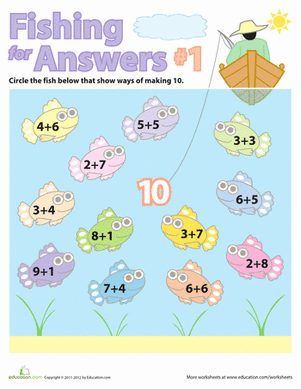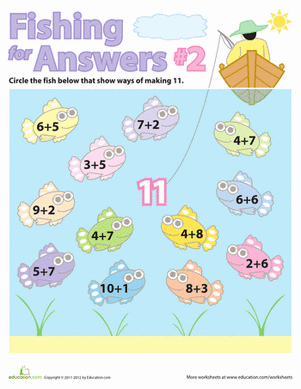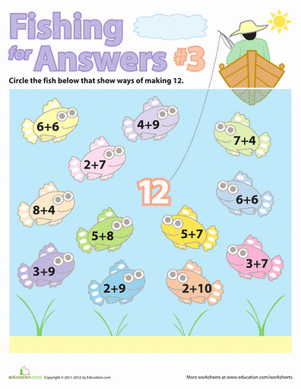There are plenty of fish in the sea! But only a few add together to make 12! This fun worksheet will help your child practise his simple addition.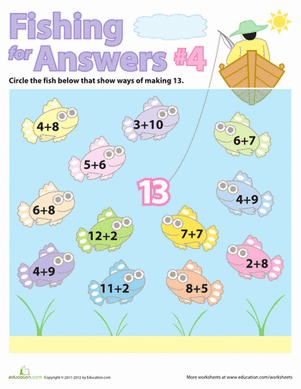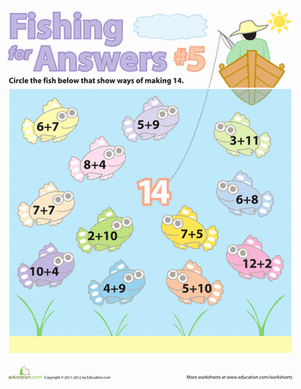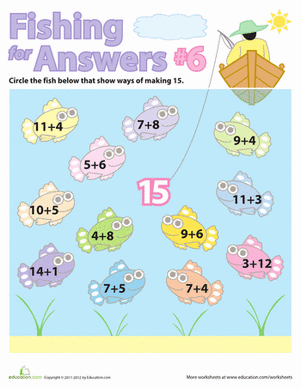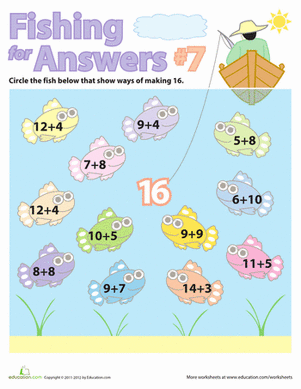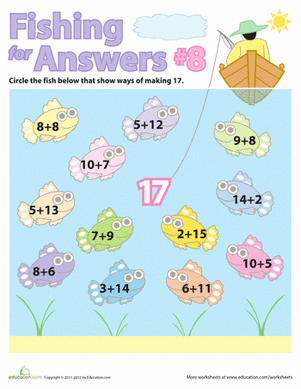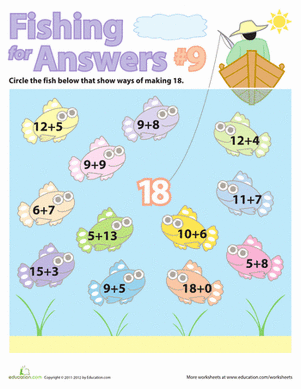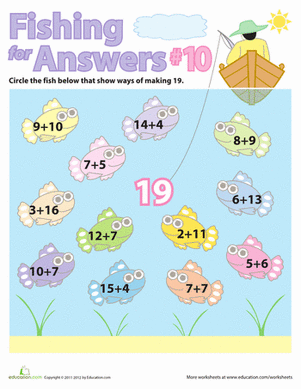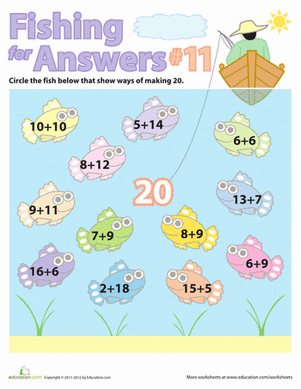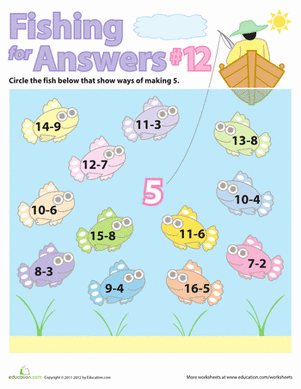Your child will get to "Fish for Answers" with this fun maths facts worksheet!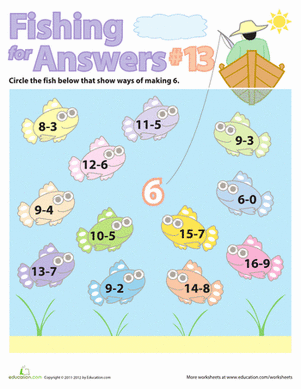Your child will get to "Fish for Answers" with this fun subtraction worksheet, a great way to practise subtraction maths facts!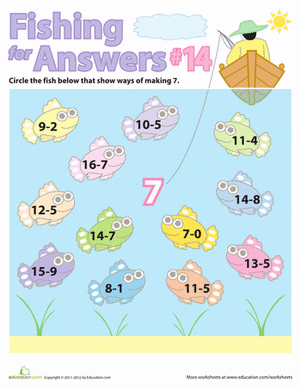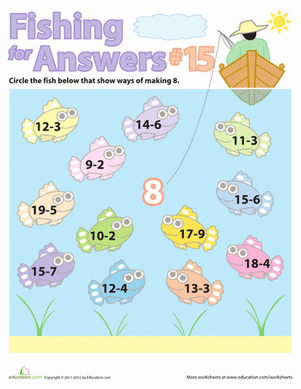Play the "Fish for Answers" game, and make subtraction seem easy! Find all the equations that equal eight.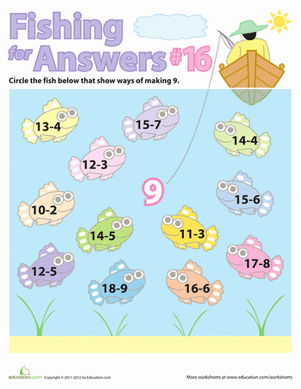Go fishing with a fun maths worksheet! Your first-grader can practise visualizing all the ways to subtract one and two digit numbers to equal nine.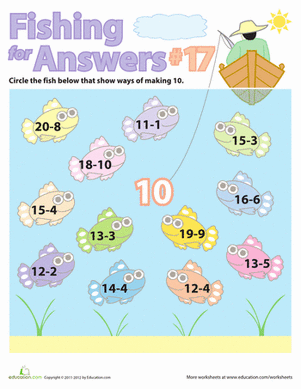Practise simple subtraction with your child. She can play the "Fish for Answers" game to learn maths facts!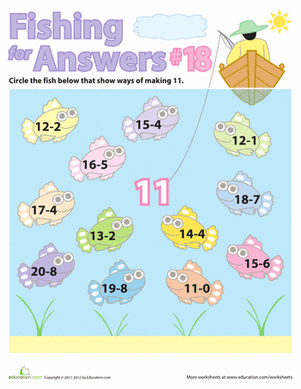If maths is becoming a bummer, just go fishing! This fun game will be perfect for introducing your first grader to simple subtraction equations.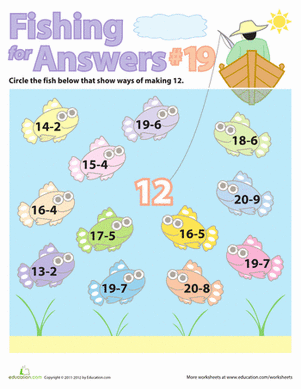Introduce your child to simple subtraction with a fun "Fish for Answers" maths game!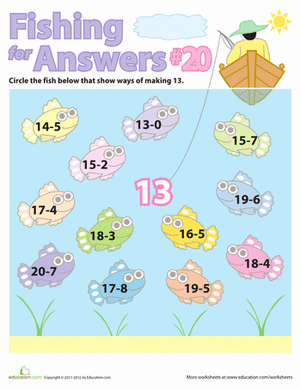Practise solving simple subtraction problems with a fun game! You and your child can "fish for answers" to find all the ways to make the number thirteen.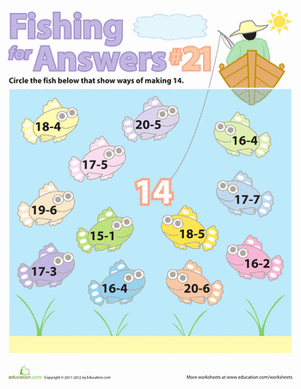You and your first grader will get to "Fish for Answers" with this fun subtraction facts worksheet.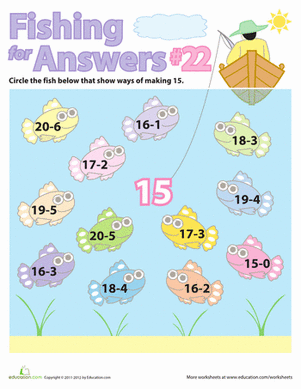Give your child a break from boring maths facts, and challenge her with a fun subtraction game!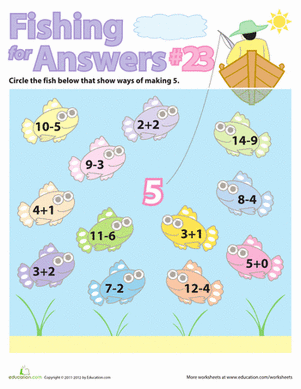Make maths fun with this series of "Fishing for Answers" maths facts!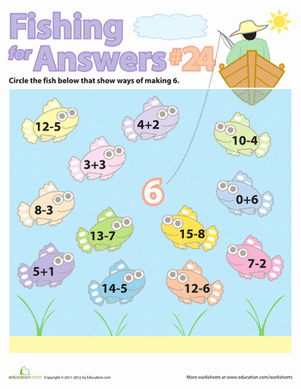Give your child a great game for practising maths facts! She'll "fish" for the right answer by solving all the simple subtraction and addition equations.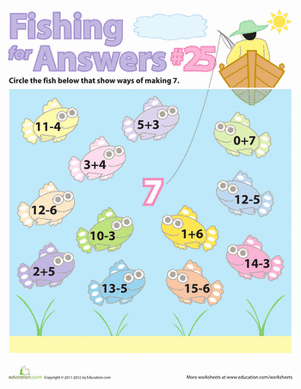Go fishing for maths facts with a fun game! Your first grader will practise solving simple addition and subtraction problems.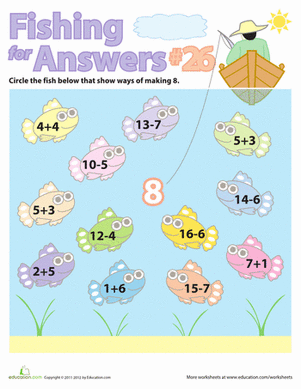Make maths fun with this series of "Fishing for Answers" addition and subtraction maths facts.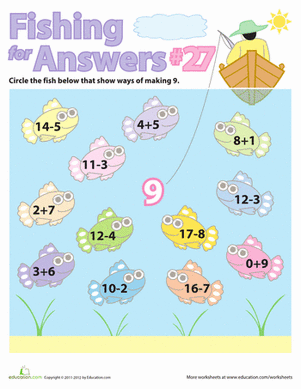Give your first grader a great visual learning game, where he can "Fish for Answers" and learn his maths facts!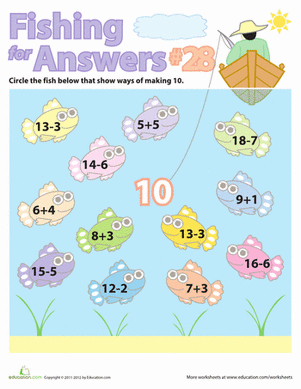Maths got your child down in the dumps? Just go fishing! Play the "Fish for Answers" game to learn simple addition and subtraction.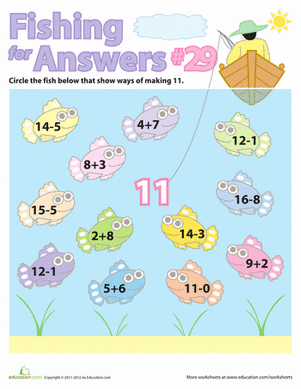Make maths facts fun with this series of "Fishing for Answers" addition and subtraction games!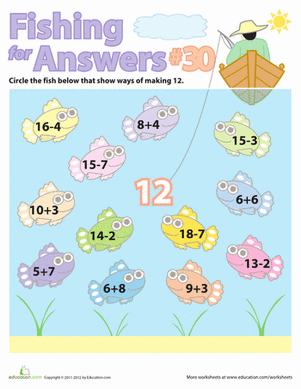How many different ways can you add and subtract to make the number twelve? Find out with a fun "Fishing for Answers" maths facts game!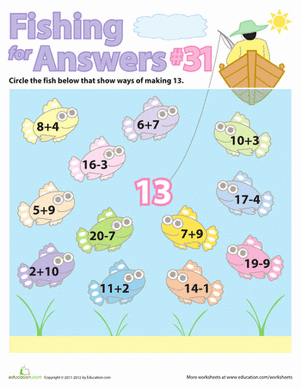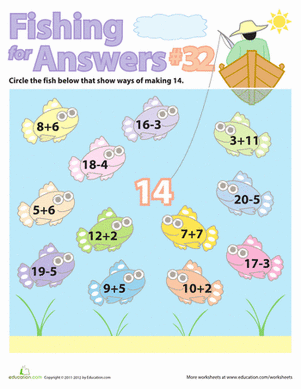Make maths fun with this series of "Fishing for Answers" maths facts. Your child will be able to practise doing simple subtraction and addition equations.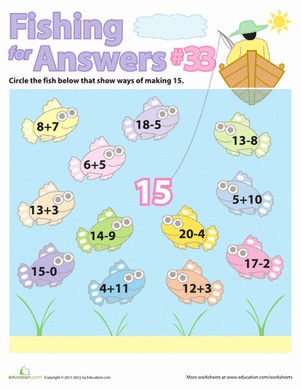Does your child need a jump-start on simple arithmetic? Give her a great game to get started learning addition and subtraction maths facts!

Create new collection

0

### New Collection>

0Items

What could we do to improve Education.com?